## Download PDF

How to read this lecture...

Code should execute sequentially if run in a Jupyter notebook

# An Introductory Example¶

## Contents¶

We’re now ready to start learning the Python language itself.

The level of this and the next few lectures will suit those with some basic knowledge of programming.

But don’t give up if you have none—you are not excluded.

You just need to cover a few of the fundamentals of programming before returning here.

Good references for first time programmers include:

Note: These references offer help on installing Python but you should probably stick with the method on our set up page.

You’ll then have an outstanding scientific computing environment (Anaconda) and be ready to move on to the rest of our course.

## Overview¶

In this lecture, we will write and then pick apart small Python programs.

The objective is to introduce you to basic Python syntax and data structures.

Deeper concepts will be covered in later lectures.

### Prerequisites¶

The lecture on getting started with Python.

## The Task: Plotting a White Noise Process¶

Suppose we want to simulate and plot the white noise process $\epsilon_0, \epsilon_1, \ldots, \epsilon_T$, where each draw $\epsilon_t$ is independent standard normal.

In other words, we want to generate figures that look something like this:We’ll do this in several different ways.

## Version 1¶

Here are a few lines of code that perform the task we set

In :
import numpy as np
import matplotlib.pyplot as plt
%matplotlib inline

x = np.random.randn(100)
plt.plot(x)
plt.show()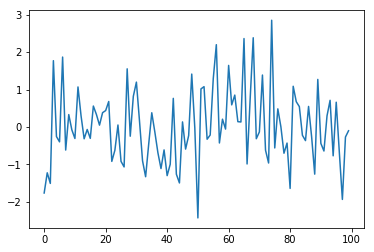Let’s break this program down and see how it works.

### Import Statements¶

The first two lines of the program import functionality.

The first line imports NumPy, a favorite Python package for tasks like

• working with arrays (vectors and matrices)
• common mathematical functions like cos and sqrt
• generating random numbers
• linear algebra, etc.

After import numpy as np we have access to these attributes via the syntax np..

Here’s another example

In :
import numpy as np

np.sqrt(4)

Out:
2.0

We could also just write

In :
import numpy

numpy.sqrt(4)

Out:
2.0

But the former method is convenient and more standard.

#### Why all the Imports?¶

Remember that Python is a general-purpose language.

The core language is quite small so it’s easy to learn and maintain.

When you want to do something interesting with Python, you almost always need to import additional functionality.

Scientific work in Python is no exception.

Most of our programs start off with lines similar to the import statements seen above.

#### Packages¶

As stated above, NumPy is a Python package.

Packages are used by developers to organize a code library.

In fact, a package is just a directory containing

1. files with Python code — called modules in Python speak
2. possibly some compiled code that can be accessed by Python (e.g., functions compiled from C or FORTRAN code)
3. a file called __init__.py that specifies what will be executed when we type import package_name

In fact, you can find and explore the directory for NumPy on your computer easily enough if you look around.

On this machine, it’s located in

anaconda3/lib/python3.6/site-packages/numpy


#### Subpackages¶

Consider the line x = np.random.randn(100).

Here np refers to the package NumPy, while random is a subpackage of NumPy.

You can see the contents here.

Subpackages are just packages that are subdirectories of another package.

### Importing Names Directly¶

Recall this code that we saw above

In :
import numpy as np

np.sqrt(4)

Out:
2.0

Here’s another way to access NumPy’s square root function

In :
from numpy import sqrt

sqrt(4)

Out:
2.0

This is also fine.

The advantage is less typing if we use sqrt often in our code.

The disadvantage is that, in a long program, these two lines might be separated by many other lines.

Then it’s harder for readers to know where sqrt came from, should they wish to.

## Alternative Versions¶

Let’s try writing some alternative versions of our first program.

Our aim in doing this is to illustrate some more Python syntax and semantics.

The programs below are less efficient but

• help us understand basic constructs like loops
• illustrate common data types like lists

### A Version with a For Loop¶

Here’s a version that illustrates loops and Python lists.

In :
ts_length = 100
ϵ_values = []   # Empty list

for i in range(ts_length):
e = np.random.randn()
ϵ_values.append(e)

plt.plot(ϵ_values)
plt.show()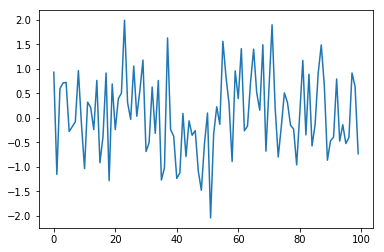In brief,

• The first pair of lines import functionality as before.
• The next line sets the desired length of the time series.
• The next line creates an empty list called ϵ_values that will store the $\epsilon_t$ values as we generate them.
• The next three lines are the for loop, which repeatedly draws a new random number $\epsilon_t$ and appends it to the end of the list ϵ_values.
• The last two lines generate the plot and display it to the user.

Let’s study some parts of this program in more detail.

### Lists¶

Consider the statement ϵ_values = [], which creates an empty list.

Lists are a native Python data structure used to group a collection of objects.

For example, try

In :
x = [10, 'foo', False]  # We can include heterogeneous data inside a list
type(x)

Out:
list

The first element of x is an integer, the next is a string and the third is a Boolean value.

When adding a value to a list, we can use the syntax list_name.append(some_value)

In :
x

Out:
[10, 'foo', False]
In :
x.append(2.5)
x

Out:
[10, 'foo', False, 2.5]

Here append() is what’s called a method, which is a function “attached to” an object—in this case, the list x.

We’ll learn all about methods later on, but just to give you some idea,

• Python objects such as lists, strings, etc. all have methods that are used to manipulate the data contained in the object.
• String objects have string methods, list objects have list methods, etc.

Another useful list method is pop()

In :
x

Out:
[10, 'foo', False, 2.5]
In :
x.pop()

Out:
2.5
In :
x

Out:
[10, 'foo', False]

The full set of list methods can be found here.

Following C, C++, Java, etc., lists in Python are zero-based

In :
x

Out:
[10, 'foo', False]
In :
x

Out:
10
In :
x

Out:
'foo'

### The For Loop¶

Now let’s consider the for loop from the program above, which was

In :
for i in range(ts_length):
e = np.random.randn()
ϵ_values.append(e)


Python executes the two indented lines ts_length times before moving on.

These two lines are called a code block, since they comprise the “block” of code that we are looping over.

Unlike most other languages, Python knows the extent of the code block only from indentation.

In our program, indentation decreases after line ϵ_values.append(e), telling Python that this line marks the lower limit of the code block.

More on indentation below—for now, let’s look at another example of a for loop

In :
animals = ['dog', 'cat', 'bird']
for animal in animals:
print("The plural of " + animal + " is " + animal + "s")

The plural of dog is dogs
The plural of cat is cats
The plural of bird is birds


This example helps to clarify how the for loop works: When we execute a loop of the form

for variable_name in sequence:
<code block>


The Python interpreter performs the following:

• For each element of the sequence, it “binds” the name variable_name to that element and then executes the code block.

The sequence object can in fact be a very general object, as we’ll see soon enough.

### Code Blocks and Indentation¶

In discussing the for loop, we explained that the code blocks being looped over are delimited by indentation.

In fact, in Python, all code blocks (i.e., those occurring inside loops, if clauses, function definitions, etc.) are delimited by indentation.

Thus, unlike most other languages, whitespace in Python code affects the output of the program.

Once you get used to it, this is a good thing: It

• forces clean, consistent indentation, improving readability
• removes clutter, such as the brackets or end statements used in other languages

On the other hand, it takes a bit of care to get right, so please remember:

• The line before the start of a code block always ends in a colon

• for i in range(10):
• if x > y:
• while x < 100:
• etc., etc.
• All lines in a code block must have the same amount of indentation.

• The Python standard is 4 spaces, and that’s what you should use.

#### Tabs vs Spaces¶

One small “gotcha” here is the mixing of tabs and spaces, which often leads to errors.

(Important: Within text files, the internal representation of tabs and spaces is not the same)

You can use your Tab key to insert 4 spaces, but you need to make sure it’s configured to do so.

If you are using a Jupyter notebook you will have no problems here.

Also, good text editors will allow you to configure the Tab key to insert spaces instead of tabs — trying searching online.

### While Loops¶

The for loop is the most common technique for iteration in Python.

But, for the purpose of illustration, let’s modify the program above to use a while loop instead.

In :
ts_length = 100
ϵ_values = []
i = 0
while i < ts_length:
e = np.random.randn()
ϵ_values.append(e)
i = i + 1
plt.plot(ϵ_values)
plt.show()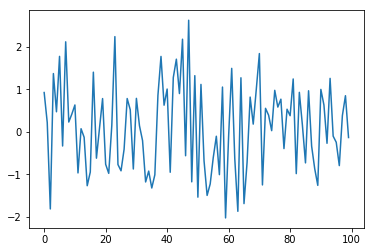Note that

• the code block for the while loop is again delimited only by indentation
• the statement i = i + 1 can be replaced by i += 1

### User-Defined Functions¶

Now let’s go back to the for loop, but restructure our program to make the logic clearer.

To this end, we will break our program into two parts:

1. A user-defined function that generates a list of random variables.
2. The main part of the program that

1. calls this function to get data
2. plots the data

This is accomplished in the next program

In :
def generate_data(n):
ϵ_values = []
for i in range(n):
e = np.random.randn()
ϵ_values.append(e)
return ϵ_values

data = generate_data(100)
plt.plot(data)
plt.show()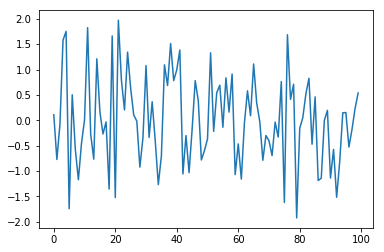Let’s go over this carefully, in case you’re not familiar with functions and how they work.

We have defined a function called generate_data() as follows

• def is a Python keyword used to start function definitions.
• def generate_data(n): indicates that the function is called generate_data and that it has a single argument n.
• The indented code is a code block called the function body—in this case, it creates an IID list of random draws using the same logic as before.
• The return keyword indicates that ϵ_values is the object that should be returned to the calling code.

This whole function definition is read by the Python interpreter and stored in memory.

When the interpreter gets to the expression generate_data(100), it executes the function body with n set equal to 100.

The net result is that the name data is bound to the list ϵ_values returned by the function.

### Conditions¶

Our function generate_data() is rather limited.

Let’s make it slightly more useful by giving it the ability to return either standard normals or uniform random variables on $(0, 1)$ as required.

This is achieved the next piece of code.

In :
def generate_data(n, generator_type):
ϵ_values = []
for i in range(n):
if generator_type == 'U':
e = np.random.uniform(0, 1)
else:
e = np.random.randn()
ϵ_values.append(e)
return ϵ_values

data = generate_data(100, 'U')
plt.plot(data)
plt.show()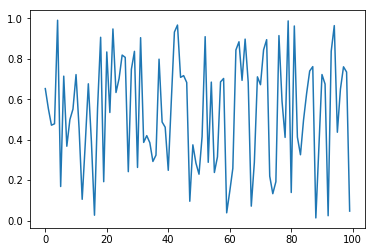Hopefully, the syntax of the if/else clause is self-explanatory, with indentation again delimiting the extent of the code blocks.

Notes

• We are passing the argument U as a string, which is why we write it as 'U'.
• Notice that equality is tested with the == syntax, not =.

• For example, the statement a = 10 assigns the name a to the value 10.
• The expression a == 10 evaluates to either True or False, depending on the value of a.

Now, there are several ways that we can simplify the code above.

For example, we can get rid of the conditionals all together by just passing the desired generator type as a function.

To understand this, consider the following version.

In :
def generate_data(n, generator_type):
ϵ_values = []
for i in range(n):
e = generator_type()
ϵ_values.append(e)
return ϵ_values

data = generate_data(100, np.random.uniform)
plt.plot(data)
plt.show()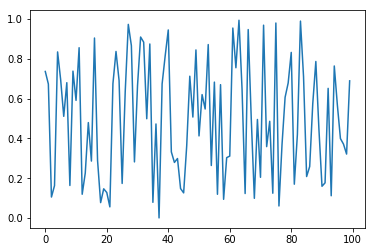Now, when we call the function generate_data(), we pass np.random.uniform as the second argument.

This object is a function.

When the function call generate_data(100, np.random.uniform) is executed, Python runs the function code block with n equal to 100 and the name generator_type “bound” to the function np.random.uniform.

• While these lines are executed, the names generator_type and np.random.uniform are “synonyms”, and can be used in identical ways.

This principle works more generally—for example, consider the following piece of code

In :
max(7, 2, 4)   # max() is a built-in Python function

Out:
7
In :
m = max
m(7, 2, 4)

Out:
7

Here we created another name for the built-in function max(), which could then be used in identical ways.

In the context of our program, the ability to bind new names to functions means that there is no problem passing a function as an argument to another function—as we did above.

### List Comprehensions¶

We can also simplify the code for generating the list of random draws considerably by using something called a list comprehension.

List comprehensions are an elegant Python tool for creating lists.

Consider the following example, where the list comprehension is on the right-hand side of the second line

In :
animals = ['dog', 'cat', 'bird']
plurals = [animal + 's' for animal in animals]
plurals

Out:
['dogs', 'cats', 'birds']

Here’s another example

In :
range(8)

Out:
range(0, 8)
In :
doubles = [2 * x for x in range(8)]
doubles

Out:
[0, 2, 4, 6, 8, 10, 12, 14]

With the list comprehension syntax, we can simplify the lines

ϵ_values = []
for i in range(n):
e = generator_type()
ϵ_values.append(e)


into

ϵ_values = [generator_type() for i in range(n)]


## Exercises¶

### Exercise 1¶

Recall that $n!$ is read as “$n$ factorial” and defined as $n! = n \times (n - 1) \times \cdots \times 2 \times 1$.

There are functions to compute this in various modules, but let’s write our own version as an exercise.

In particular, write a function factorial such that factorial(n) returns $n!$ for any positive integer $n$.

### Exercise 2¶

The binomial random variable $Y \sim Bin(n, p)$ represents the number of successes in $n$ binary trials, where each trial succeeds with probability $p$.

Without any import besides from numpy.random import uniform, write a function binomial_rv such that binomial_rv(n, p) generates one draw of $Y$.

Hint: If $U$ is uniform on $(0, 1)$ and $p \in (0,1)$, then the expression U < p evaluates to True with probability $p$.

### Exercise 3¶

Compute an approximation to $\pi$ using Monte Carlo. Use no imports besides

In :
import numpy as np


Your hints are as follows:

• If $U$ is a bivariate uniform random variable on the unit square $(0, 1)^2$, then the probability that $U$ lies in a subset $B$ of $(0,1)^2$ is equal to the area of $B$.
• If $U_1,\ldots,U_n$ are IID copies of $U$, then, as $n$ gets large, the fraction that falls in $B$, converges to the probability of landing in $B$.
• For a circle, area = pi * radius^2.

### Exercise 4¶

Write a program that prints one realization of the following random device:

• Flip an unbiased coin 10 times.
• If 3 consecutive heads occur one or more times within this sequence, pay one dollar.
• If not, pay nothing.

Use no import besides from numpy.random import uniform.

### Exercise 5¶

Your next task is to simulate and plot the correlated time series

$$x_{t+1} = \alpha \, x_t + \epsilon_{t+1} \quad \text{where} \quad x_0 = 0 \quad \text{and} \quad t = 0,\ldots,T$$

The sequence of shocks $\{\epsilon_t\}$ is assumed to be IID and standard normal.

In your solution, restrict your import statements to

In :
import numpy as np
import matplotlib.pyplot as plt


Set $T=200$ and $\alpha = 0.9$.

### Exercise 6¶

To do the next exercise, you will need to know how to produce a plot legend.

The following example should be sufficient to convey the idea

In :
import numpy as np
import matplotlib.pyplot as plt

x = [np.random.randn() for i in range(100)]
plt.plot(x, label="white noise")
plt.legend()
plt.show()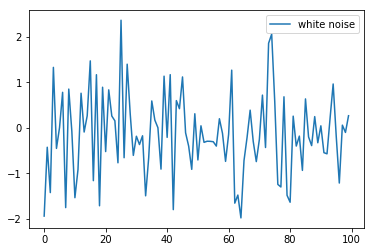Now, starting with your solution to exercise 5, plot three simulated time series, one for each of the cases $\alpha=0$, $\alpha=0.8$ and $\alpha=0.98$.

In particular, you should produce (modulo randomness) a figure that looks as follows(The figure nicely illustrates how time series with the same one-step-ahead conditional volatilities, as these three processes have, can have very different unconditional volatilities.)

Use a for loop to step through the $\alpha$ values.

Important hints:

• If you call the plot() function multiple times before calling show(), all of the lines you produce will end up on the same figure.

• And if you omit the argument 'b-' to the plot function, Matplotlib will automatically select different colors for each line.
• The expression 'foo' + str(42) evaluates to 'foo42'.

## Solutions¶

### Exercise 1¶

In :
def factorial(n):
k = 1
for i in range(n):
k = k * (i + 1)
return k

factorial(4)

Out:
24

### Exercise 2¶

In :
from numpy.random import uniform

def binomial_rv(n, p):
count = 0
for i in range(n):
U = uniform()
if U < p:
count = count + 1    # Or count += 1
return count

binomial_rv(10, 0.5)

Out:
5

### Exercise 3¶

Consider the circle of diameter 1 embedded in the unit square.

Let $A$ be its area and let $r=1/2$ be its radius.

If we know $\pi$ then we can compute $A$ via $A = \pi r^2$.

But here the point is to compute $\pi$, which we can do by $\pi = A / r^2$.

Summary: If we can estimate the area of the unit circle, then dividing by $r^2 = (1/2)^2 = 1/4$ gives an estimate of $\pi$.

We estimate the area by sampling bivariate uniforms and looking at the fraction that falls into the unit circle

In :
n = 100000

count = 0
for i in range(n):
u, v = np.random.uniform(), np.random.uniform()
d = np.sqrt((u - 0.5)**2 + (v - 0.5)**2)
if d < 0.5:
count += 1

area_estimate = count / n

print(area_estimate * 4)  # dividing by radius**2

3.14228


### Exercise 4¶

In :
from numpy.random import uniform

payoff = 0
count = 0

for i in range(10):
U = uniform()
count = count + 1 if U < 0.5 else 0
if count == 3:
payoff = 1

print(payoff)

0


### Exercise 5¶

The next line embeds all subsequent figures in the browser itself

In :
α = 0.9
ts_length = 200
current_x = 0

x_values = []
for i in range(ts_length + 1):
x_values.append(current_x)
current_x = α * current_x + np.random.randn()
plt.plot(x_values)
plt.show()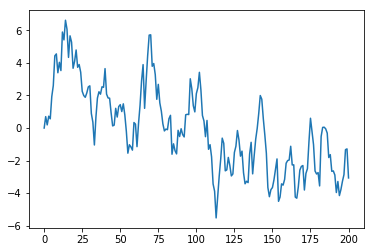### Exercise 6¶

In :
αs = [0.0, 0.8, 0.98]
ts_length = 200

for α in αs:
x_values = []
current_x = 0
for i in range(ts_length):
x_values.append(current_x)
current_x = α * current_x + np.random.randn()
plt.plot(x_values, label=f'α = {α}')
plt.legend()
plt.show()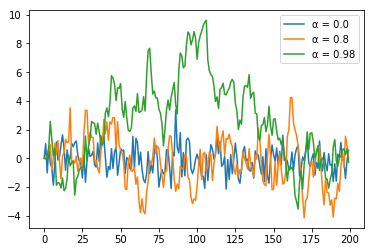• Share page